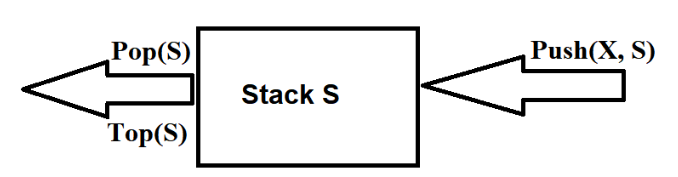# 常见的基本数据结构——栈```struct Node；
typedef struct Node *PtrTONode;
typedef PtrToNode Stack;

struct Node{
ElementType Node;
PtrToNode Next;
};```

```int

IsEmpty(Stack S){

return S->Next == NULL;

}```

```Stack
CreateStack(void){
Stack S;
S = malloc(sizeof(struct Node));
if(S == NULL){
printf(” out of space”);
}
S->Next == NULL;
MakeEmpty(S);
return S;
}
void
MakeEmpty(Stack S){
if(S == NULL){
Error();
}else{
while(!IsEmpty(S)){
Pop(s);
}
}```

Push进栈例程

```void
Push(ElememtType X, Stack S){
PtrToNode TemCell;
TemCell = malloc(sizeof(struct Node));
if(TemCell == NULL){
Error();
}else{
TemCell->ElementType = X;
TemCell->Next = S->Next;
S->Next = TemCell;
}
}```

Pop操作实现

```ElementType
Top(Stack S){
if(!IsEmpty(S))
return S->Next->Element;
Error();
return 0;
}```

```struct StackRecord；
tepedef struct StructRecord * Stack;

struct StackRecord{
int Capacity;
int TopOfStack;
int ElementType *Array;
}```
```Stack
CreateStack(int MaxElement){
Stack S;
if(MaxElement < MinStackSize)
Error();
S = malloc(sizeof(struct StackRecord));
if(S == NULL)
Error();
S->Array = malloc(sizeof(ElementType) * MaxElement);
if(S->Array == NULL)
Error();
S->Capacity = MaxElements;
MakeEmpty(S);
return S;
}```

```int
IsEmpty(Stack S){
return S->TopOfStack == EmptyTOS;
}```

```void
MakeEmpty(Stack S){
S->TopOfStack = EmptyTOS;
}```

```void
Push(ElementType S, Stack S){
if(IsFull(S))
Error();
else
S->Array[++S->TopOfStack] = X;
}```

```ElementType
Top(Stack S){
if(!IsEmpty(S))
return S->Array[S->TopOfStack];
Error();
return 0;
}```

```void
Pop(Stack S){
if(IsEmpty)
Error();
else
S-TopOfStack—;
}```

```ElementType
TopAndPop(Stack S){
if(!IsEmpty(S)){
return S->Array[S->TopOfStack];
}
Error();
return 0;
}```

4.99 + 5.99 + 6.99 * 1.06 = 18.69

4.99 1.06 * 5.99 +6.99 1.06 * +

a + b * c + (d * e + f) * g

a b c * + d e * f + g * +

```void
PrintList(List L){
if(L != NULL){
PrintElement(L->Element);
PrintList(L->Next);
}
}```

```void
PrintList(List L){
top:
if(L != NULL){
PrintElement(L->Element);
L = L->Next;
goto top;
}
}```